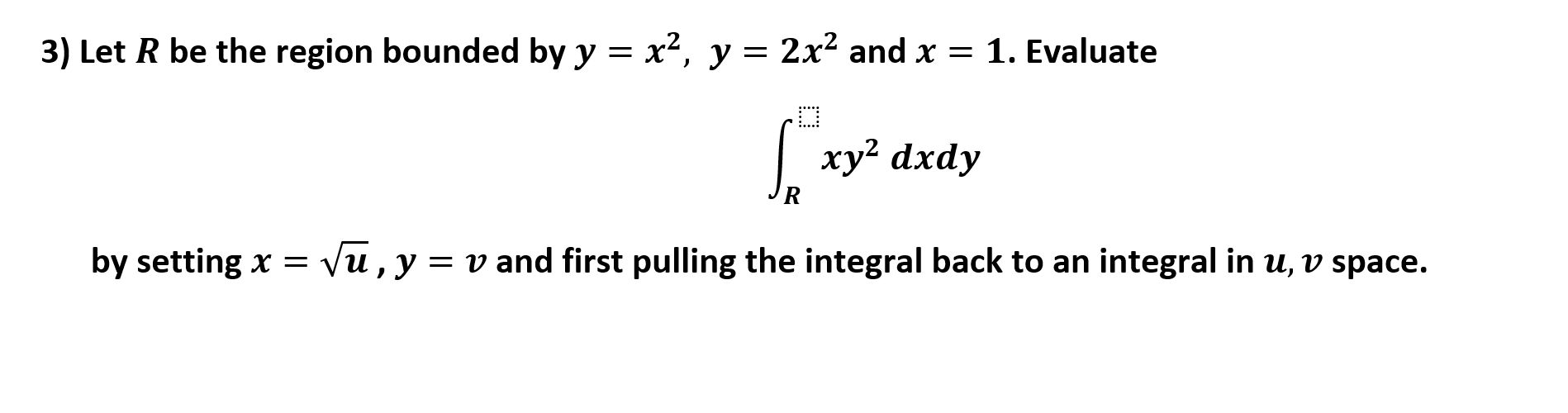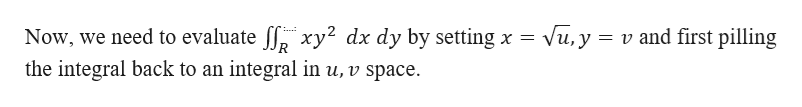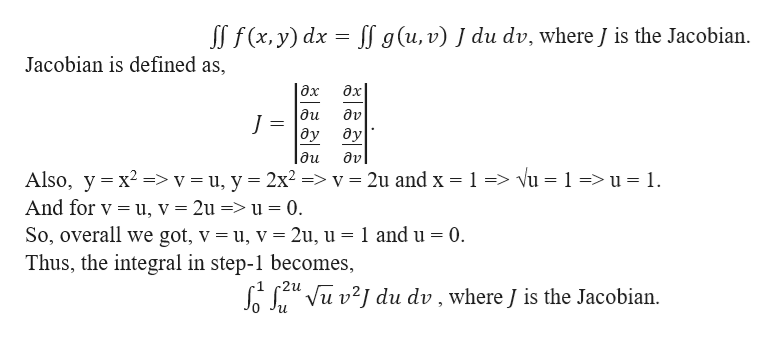# = x2, y = 2x2 and x = 1. Evaluate3) Let R be the region bounded by yxy2 dxdyVu, yv and first pulling the integral back to an integral in u, v spaceby setting x

Question
12 views

Need help integrating attached pullback pleasehelp_outlineImage Transcriptionclose= x2, y = 2x2 and x = 1. Evaluate 3) Let R be the region bounded by y xy2 dxdy Vu, y v and first pulling the integral back to an integral in u, v space by setting x fullscreen
check_circle

star
star
star
star
star
1 Rating
Step 1

Let R be the region bounded by y = x2 and y = 2x2 and x = 1.help_outlineImage TranscriptioncloseNow, we need to evaluate ff xy2 dx dy by setting x the integral back to an integral in u, v space. Vu, y v and first pilling fullscreen
Step 2

We know th...help_outlineImage TranscriptioncloseSS f(x, y) dx I g (u, v) J du dv, where J is the Jacobian Jacobian is defined as, |дх дх ди J ду ду дu av Also, y x2v = u, y 2x2 ->v - 2u and x 1> vu = 1 => u = 1. And for v u, v = 2u => u = 0 So, overall we got, v = u, v = 2u, u = 1 and u = 0. Thus, the integral in step-1 becomes (z" Vu v2/ du dv , where J is the Jacobian. fullscreen

### Want to see the full answer?

See Solution

#### Want to see this answer and more?

Solutions are written by subject experts who are available 24/7. Questions are typically answered within 1 hour.*

See Solution
*Response times may vary by subject and question.
Tagged in

### Math